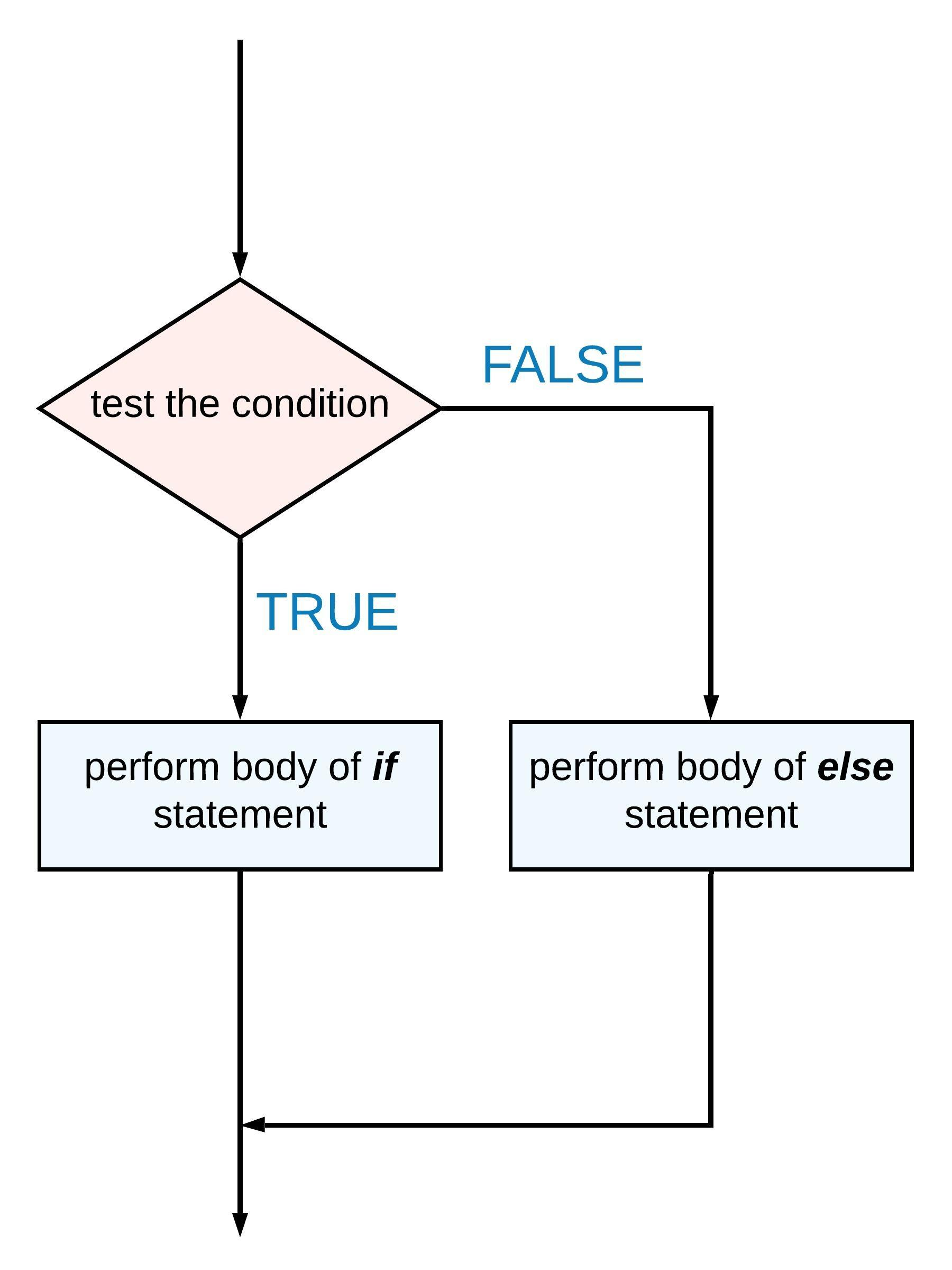Often in our code, decisions will have to be made. If/else statements handle this. The idea is very simple; if a test condition has been met, “do one thing”, else “do the other”. It “looks like” this:The R code structure is also very simple. In R, if/else statements are written like this:

if( logical condition ){

perform these instructions

}else{

perform these alternate instructions

}

Notice that the instructions are held within curly brackets, { } just like the instructions for defining a function.

Here are some examples of common “conditions” and how they are written:

condition description
( x == a ) == exactly equal to $$a$$ (note the double equals)
( x <= a ) <= less than or equal to $$a$$
( x >= a ) >= greater than or equal to $$a$$
( x != a ) != not equal to $$a$$
((x < a ) | ( x > b )) | or
((x > a ) & ( x < b )) & and

#### example 1

Here’s a simple if/else statement to respond to the sign of a number:

x <- -2

if(x >= 0) {
print("x is positive")
}else{
print("x is negative")
}
##  "x is negative"

#### example 2

Let’s now improve our quadratic solver.

solve.quadratic <- function(a, b, c) {

D <- b ^ 2 - 4 * a * c

if (D >= 0) {
x1 <- (-b - sqrt(D)) / (2 * a)
x2 <- (-b + sqrt(D)) / (2 * a)
v <- c(x1, x2)
return(v)
}else{
print("no real roots")
}
}

solve.quadratic(1,1,1)
##  "no real roots"
solve.quadratic(1,1,-6)
##  -3  2

Now, the code will only calculate the roots if they are real.

#### example 3

In a simple dice game, you roll two dice. You win if the sum of the throws equals $$6$$. Write a function which simulates this game and lets you know if the game resulted in a win or a loss.

We will use the function sample() which can be used to pick an element at random from a vector.

dice.game <- function(){

D <- 1:6
#the following code randomly picks 1 number from D = {1,2,3,4,5,6}
throw.1 <- sample(D, 1)
throw.2 <- sample(D, 1)

result <- throw.1 + throw.2

if( result == 6 ) {
print(c(throw.1, throw.2, "you win!"))
}else{
print(c(throw.1, throw.2, "you lose!"))
}
}

dice.game()
##  "1"         "3"         "you lose!"
dice.game()
##  "2"         "6"         "you lose!"
dice.game()
##  "2"         "2"         "you lose!"
dice.game()
##  "5"        "1"        "you win!"